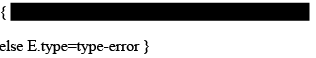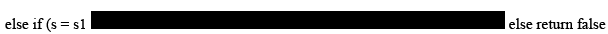Courses

# Type Checking & Type Expressions - Intermediate Code Generation Computer Science Engineering (CSE) Notes | EduRev

## Computer Science Engineering (CSE) : Type Checking & Type Expressions - Intermediate Code Generation Computer Science Engineering (CSE) Notes | EduRev

The document Type Checking & Type Expressions - Intermediate Code Generation Computer Science Engineering (CSE) Notes | EduRev is a part of the Computer Science Engineering (CSE) Course Compiler Design.
All you need of Computer Science Engineering (CSE) at this link: Computer Science Engineering (CSE)

Type Expression:
•The type of a language construct is denoted by a type expression.

•A type expression can be:

–A basic type

•a primitive data type such as integer, real, char, Boolean, …

•type-error to signal a type error

•void: no type

–A type name

•a name can be used to denote a type expression.

–A type constructor applies to other type expressions.

•arrays: If T is a type expression, then array (I,T)is a type expression where I denotes index range. Ex: array (0..99,int)

•products: If T1and T2 are type expressions, then their Cartesian product T1 x T2 is a type expression. Ex: int x int

•pointers: If T is a type expression, then pointer (T) is a type expression. Ex: pointer (int)

•functions: We may treat functions in a programming language as mapping from a domain type D to a range type R. So, the type of a function can be denoted by the type expression D→R where D are R type expressions. Ex: int→int represents the type of a function which takes an int value as parameter, and its return type is also int.

Type Checking of Statements: S ->d= E

{ if (id.type=E.type then S.type=void
else S.type=type-error }

S ->if E then S1
{ if (E.type=boolean then S.type=S1.type
else S.type=type-error }
S->while E do S1
{ if (E.type=boolean then S.type=S1.type
else S.type=type-error }

Type Checking of Functions:
E->E1( E2)Ex: int f(double x, char y) { ... }
f:                    double x char->int argume types return type

Structural Equivalence of Type Expressions:

•How do we know that two type expressions are equal?

•As long as type expressions are built from basic types (no type names), we may use structural equivalence between two type expressions

Structural Equivalence Algorithm (sequin):

if (s and t are same basic types) then return true

else if (s=array(s1,s2) and t=array(t1,t2)) then return (sequiv(s1,t1) and sequiv(s2,t2)) else if (s = s1 x

s2and t = t1 x t2) then return (sequiv(s1,t1) and sequiv(s2,t2))

else if (s=pointer(s1) and t=pointer(t1)) then return (sequiv(s1,t1))Names for Type Expressions:

•In some programming languages, we give a name to a type expression, and we use that name as a type expression afterwards.

type link = ↑cell; ? p,q,r,s have same types ? var p,q : link;
var r,s : ↑cell

•How do we treat type names?

–Get equivalent type expression for a type name (then use structural equivalence), or

–Treat a type name as a basic type

Offer running on EduRev: Apply code STAYHOME200 to get INR 200 off on our premium plan EduRev Infinity!

## Compiler Design

16 videos|44 docs|29 tests

,

,

,

,

,

,

,

,

,

,

,

,

,

,

,

,

,

,

,

,

,

;